## 3.1 The Mole and Molar Masses

### Learning Objective

1. To calculate the molecular mass of a covalent compound and the formula mass of an ionic compound and to calculate the number of atoms, molecules, or formula units in a sample of a substance.

As you learned in Chapter 1 "Introduction to Chemistry", the mass number is the sum of the numbers of protons and neutrons present in the nucleus of an atom. The mass number is an integer that is approximately equal to the numerical value of the atomic mass. Although the mass number is unitless, it is assigned units called atomic mass units (amu). Because a molecule or a polyatomic ion is an assembly of atoms whose identities are given in its molecular or ionic formula, we can calculate the average atomic mass of any molecule or polyatomic ion from its composition by adding together the masses of the constituent atoms. The average mass of a monatomic ion is the same as the average mass of an atom of the element because the mass of electrons is so small that it is insignificant in most calculations.

## Molecular and Formula Masses

The molecular massThe sum of the average masses of the atoms in one molecule of a substance, each multiplied by its subscript. of a substance is the sum of the average masses of the atoms in one molecule of a substance. It is calculated by adding together the atomic masses of the elements in the substance, each multiplied by its subscript (written or implied) in the molecular formula. Because the units of atomic mass are atomic mass units, the units of molecular mass are also atomic mass units. The procedure for calculating molecular masses is illustrated in Example 1.

### Example 1

Calculate the molecular mass of ethanol, whose condensed structural formula is CH3CH2OH. Among its many uses, ethanol is a fuel for internal combustion engines.

Given: molecule

Strategy:

A Determine the number of atoms of each element in the molecule.

B Obtain the atomic masses of each element from the periodic table and multiply the atomic mass of each element by the number of atoms of that element.

C Add together the masses to give the molecular mass.

Solution:

A The molecular formula of ethanol may be written in three different ways: CH3CH2OH (which illustrates the presence of an ethyl group, CH3CH2−, and an −OH group), C2H5OH, and C2H6O; all show that ethanol has two carbon atoms, six hydrogen atoms, and one oxygen atom.

B Taking the atomic masses from the periodic table, we obtain

C Adding together the masses gives the molecular mass:

24.022 amu + 6.0474 amu + 15.9994 amu = 46.069 amu

Alternatively, we could have used unit conversions to reach the result in one step, as described in Essential Skills 2 (Section 3.7 "Essential Skills 2"):

The same calculation can also be done in a tabular format, which is especially helpful for more complex molecules:

Exercise

Calculate the molecular mass of trichlorofluoromethane, also known as Freon-11, whose condensed structural formula is CCl3F. Until recently, it was used as a refrigerant. The structure of a molecule of Freon-11 is as follows: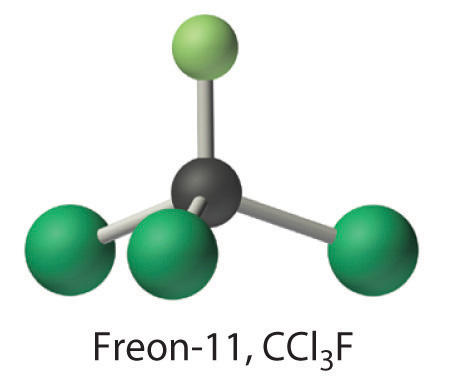Unlike molecules, which have covalent bonds, ionic compounds do not have a readily identifiable molecular unit. So for ionic compounds we use the formula mass (also called the empirical formula massAnother name for formula mass.) of the compound rather than the molecular mass. The formula massThe sum of the atomic masses of all the elements in the empirical formula, each multiplied by its subscript. is the sum of the atomic masses of all the elements in the empirical formula, each multiplied by its subscript (written or implied). It is directly analogous to the molecular mass of a covalent compound. Once again, the units are atomic mass units.

### Note the Pattern

Atomic mass, molecular mass, and formula mass all have the same units: atomic mass units.

### Example 2

Calculate the formula mass of Ca3(PO4)2, commonly called calcium phosphate. This compound is the principal source of calcium found in bovine milk.

Given: ionic compound

Strategy:

A Determine the number of atoms of each element in the empirical formula.

B Obtain the atomic masses of each element from the periodic table and multiply the atomic mass of each element by the number of atoms of that element.

C Add together the masses to give the formula mass.

Solution:

A The empirical formula—Ca3(PO4)2—indicates that the simplest electrically neutral unit of calcium phosphate contains three Ca2+ ions and two PO43− ions. The formula mass of this molecular unit is calculated by adding together the atomic masses of three calcium atoms, two phosphorus atoms, and eight oxygen atoms.

B Taking atomic masses from the periodic table, we obtain

C Adding together the masses gives the formula mass of Ca3(PO4)2:

120.234 amu + 61.947522 amu + 127.9952 amu = 310.177 amu

We could also find the formula mass of Ca3(PO4)2 in one step by using unit conversions or a tabular format:

Exercise

Calculate the formula mass of Si3N4, commonly called silicon nitride. It is an extremely hard and inert material that is used to make cutting tools for machining hard metal alloys.

## The Mole

In Chapter 1 "Introduction to Chemistry", we described Dalton’s theory that each chemical compound has a particular combination of atoms and that the ratios of the numbers of atoms of the elements present are usually small whole numbers. We also described the law of multiple proportions, which states that the ratios of the masses of elements that form a series of compounds are small whole numbers. The problem for Dalton and other early chemists was to discover the quantitative relationship between the number of atoms in a chemical substance and its mass. Because the masses of individual atoms are so minuscule (on the order of 10−23 g/atom), chemists do not measure the mass of individual atoms or molecules. In the laboratory, for example, the masses of compounds and elements used by chemists typically range from milligrams to grams, while in industry, chemicals are bought and sold in kilograms and tons. To analyze the transformations that occur between individual atoms or molecules in a chemical reactionA process in which a substance is converted to one or more other substances with different compositions and properties., it is therefore absolutely essential for chemists to know how many atoms or molecules are contained in a measurable quantity in the laboratory—a given mass of sample. The unit that provides this link is the mole (mol)The quantity of a substance that contains the same number of units (e.g., atoms or molecules) as the number of carbon atoms in exactly 12 g of isotopically pure carbon-12., from the Latin moles, meaning “pile” or “heap” (not from the small subterranean animal!).

Many familiar items are sold in numerical quantities that have unusual names. For example, cans of soda come in a six-pack, eggs are sold by the dozen (12), and pencils often come in a gross (12 dozen, or 144). Sheets of printer paper are packaged in reams of 500, a seemingly large number. Atoms are so small, however, that even 500 atoms are too small to see or measure by most common techniques. Any readily measurable mass of an element or compound contains an extraordinarily large number of atoms, molecules, or ions, so an extraordinarily large numerical unit is needed to count them. The mole is used for this purpose.

A mole is defined as the amount of a substance that contains the number of carbon atoms in exactly 12 g of isotopically pure carbon-12. According to the most recent experimental measurements, this mass of carbon-12 contains 6.022142 × 1023 atoms, but for most purposes 6.022 × 1023 provides an adequate number of significant figures. Just as 1 mol of atoms contains 6.022 × 1023 atoms, 1 mol of eggs contains 6.022 × 1023 eggs. The number in a mole is called Avogadro’s numberThe number of units (e.g., atoms, molecules, or formula units) in 1 mol: $6.022×1023$., after the 19th-century Italian scientist who first proposed a relationship between the volumes of gases and the numbers of particles they contain.

It is not obvious why eggs come in dozens rather than 10s or 14s, or why a ream of paper contains 500 sheets rather than 400 or 600. The definition of a mole—that is, the decision to base it on 12 g of carbon-12—is also arbitrary. The important point is that 1 mol of carbon—or of anything else, whether atoms, compact discs, or houses—always has the same number of objects: 6.022 × 1023.

### Note the Pattern

One mole always has the same number of objects: 6.022 × 1023.

To appreciate the magnitude of Avogadro’s number, consider a mole of pennies. Stacked vertically, a mole of pennies would be 4.5 × 1017 mi high, or almost six times the diameter of the Milky Way galaxy. If a mole of pennies were distributed equally among the entire population on Earth, each person would get more than one trillion dollars. Clearly, the mole is so large that it is useful only for measuring very small objects, such as atoms.

The concept of the mole allows us to count a specific number of individual atoms and molecules by weighing measurable quantities of elements and compounds. To obtain 1 mol of carbon-12 atoms, we would weigh out 12 g of isotopically pure carbon-12. Because each element has a different atomic mass, however, a mole of each element has a different mass, even though it contains the same number of atoms (6.022 × 1023). This is analogous to the fact that a dozen extra large eggs weighs more than a dozen small eggs, or that the total weight of 50 adult humans is greater than the total weight of 50 children. Because of the way in which the mole is defined, for every element the number of grams in a mole is the same as the number of atomic mass units in the atomic mass of the element. For example, the mass of 1 mol of magnesium (atomic mass = 24.305 amu) is 24.305 g. Because the atomic mass of magnesium (24.305 amu) is slightly more than twice that of a carbon-12 atom (12 amu), the mass of 1 mol of magnesium atoms (24.305 g) is slightly more than twice that of 1 mol of carbon-12 (12 g). Similarly, the mass of 1 mol of helium (atomic mass = 4.002602 amu) is 4.002602 g, which is about one-third that of 1 mol of carbon-12. Using the concept of the mole, we can now restate Dalton’s theory: 1 mol of a compound is formed by combining elements in amounts whose mole ratios are small whole numbers. For example, 1 mol of water (H2O) has 2 mol of hydrogen atoms and 1 mol of oxygen atoms.

## Molar Mass

The molar massThe mass in grams of 1 mol of a substance. of a substance is defined as the mass in grams of 1 mol of that substance. One mole of isotopically pure carbon-12 has a mass of 12 g. For an element, the molar mass is the mass of 1 mol of atoms of that element; for a covalent molecular compound, it is the mass of 1 mol of molecules of that compound; for an ionic compound, it is the mass of 1 mol of formula units. That is, the molar mass of a substance is the mass (in grams per mole) of 6.022 × 1023 atoms, molecules, or formula units of that substance. In each case, the number of grams in 1 mol is the same as the number of atomic mass units that describe the atomic mass, the molecular mass, or the formula mass, respectively.

### Note the Pattern

The molar mass of any substance is its atomic mass, molecular mass, or formula mass in grams per mole.

The periodic table lists the atomic mass of carbon as 12.011 amu; the average molar mass of carbon—the mass of 6.022 × 1023 carbon atoms—is therefore 12.011 g/mol:

Substance (formula) Atomic, Molecular, or Formula Mass (amu) Molar Mass (g/mol)
carbon (C) 12.011 (atomic mass) 12.011
ethanol (C2H5OH) 46.069 (molecular mass) 46.069
calcium phosphate [Ca3(PO4)2] 310.177 (formula mass) 310.177

The molar mass of naturally occurring carbon is different from that of carbon-12 and is not an integer because carbon occurs as a mixture of carbon-12, carbon-13, and carbon-14. One mole of carbon still has 6.022 × 1023 carbon atoms, but 98.89% of those atoms are carbon-12, 1.11% are carbon-13, and a trace (about 1 atom in 1012) are carbon-14. (For more information, see Section 1.6 "Isotopes and Atomic Masses".) Similarly, the molar mass of uranium is 238.03 g/mol, and the molar mass of iodine is 126.90 g/mol. When we deal with elements such as iodine and sulfur, which occur as a diatomic molecule (I2) and a polyatomic molecule (S8), respectively, molar mass usually refers to the mass of 1 mol of atoms of the element—in this case I and S, not to the mass of 1 mol of molecules of the element (I2 and S8).

The molar mass of ethanol is the mass of ethanol (C2H5OH) that contains 6.022 × 1023 ethanol molecules. As you calculated in Example 1, the molecular mass of ethanol is 46.069 amu. Because 1 mol of ethanol contains 2 mol of carbon atoms (2 × 12.011 g), 6 mol of hydrogen atoms (6 × 1.0079 g), and 1 mol of oxygen atoms (1 × 15.9994 g), its molar mass is 46.069 g/mol. Similarly, the formula mass of calcium phosphate [Ca3(PO4)2] is 310.177 amu, so its molar mass is 310.177 g/mol. This is the mass of calcium phosphate that contains 6.022 × 1023 formula units. Figure 3.1 "Samples of 1 Mol of Some Common Substances" shows samples that contain precisely one molar mass of several common substances.

Figure 3.1 Samples of 1 Mol of Some Common SubstancesThe mole is the basis of quantitative chemistry. It provides chemists with a way to convert easily between the mass of a substance and the number of individual atoms, molecules, or formula units of that substance. Conversely, it enables chemists to calculate the mass of a substance needed to obtain a desired number of atoms, molecules, or formula units. For example, to convert moles of a substance to mass, we use the relationship

Equation 3.1

(moles)(molar mass) → mass

or, more specifically,

Conversely, to convert the mass of a substance to moles, we use

Equation 3.2

Be sure to pay attention to the units when converting between mass and moles.

Figure 3.2 "A Flowchart for Converting between Mass; the Number of Moles; and the Number of Atoms, Molecules, or Formula Units" is a flowchart for converting between mass; the number of moles; and the number of atoms, molecules, or formula units. The use of these conversions is illustrated in Example 3 and Example 4.

Figure 3.2 A Flowchart for Converting between Mass; the Number of Moles; and the Number of Atoms, Molecules, or Formula Units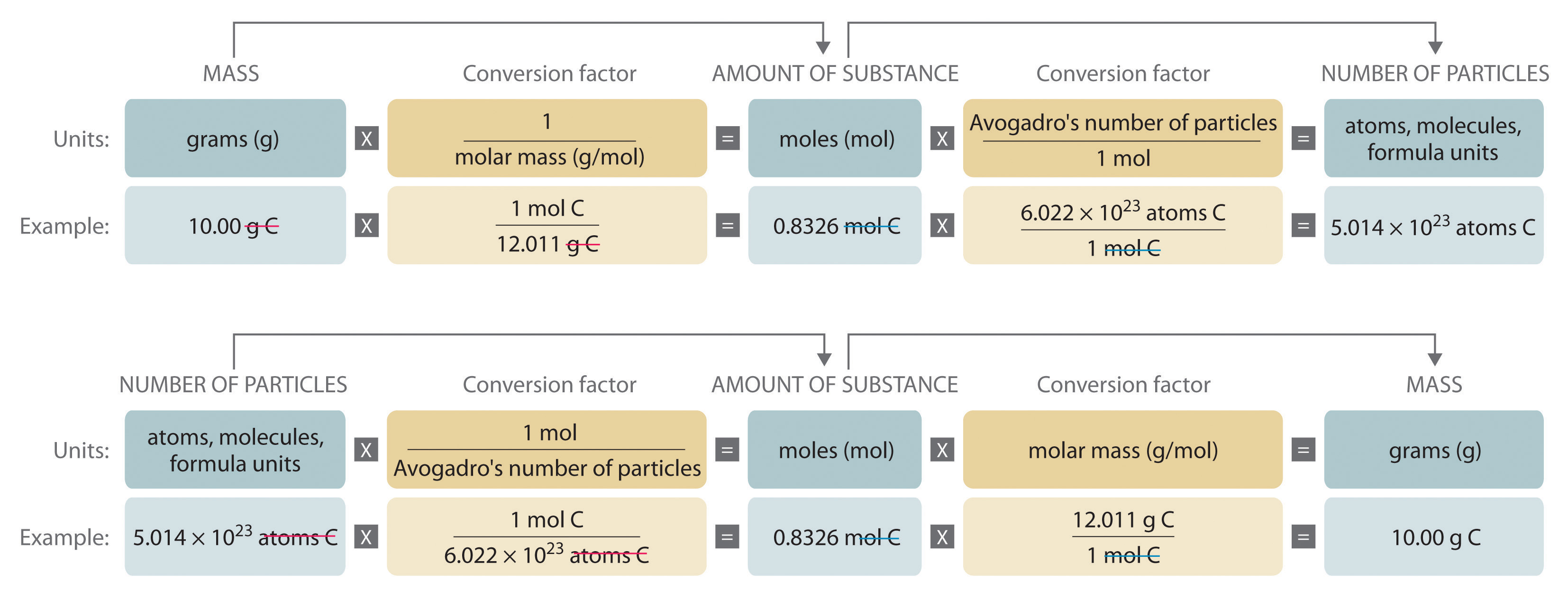### Example 3

For 35.00 g of ethylene glycol (HOCH2CH2OH), which is used in inks for ballpoint pens, calculate the number of

1. moles.
2. molecules.

Given: mass and molecular formula

Asked for: number of moles and number of molecules

Strategy:

A Use the molecular formula of the compound to calculate its molecular mass in grams per mole.

B Convert from mass to moles by dividing the mass given by the compound’s molar mass.

C Convert from moles to molecules by multiplying the number of moles by Avogadro’s number.

Solution:

1. A The molecular mass of ethylene glycol can be calculated from its molecular formula using the method illustrated in Example 1:

The molar mass of ethylene glycol is 62.068 g/mol.

B The number of moles of ethylene glycol present in 35.00 g can be calculated by dividing the mass (in grams) by the molar mass (in grams per mole):

So

It is always a good idea to estimate the answer before you do the actual calculation. In this case, the mass given (35.00 g) is less than the molar mass, so the answer should be less than 1 mol. The calculated answer (0.5639 mol) is indeed less than 1 mol, so we have probably not made a major error in the calculations.

2. C To calculate the number of molecules in the sample, we multiply the number of moles by Avogadro’s number:

Because we are dealing with slightly more than 0.5 mol of ethylene glycol, we expect the number of molecules present to be slightly more than one-half of Avogadro’s number, or slightly more than 3 × 1023 molecules, which is indeed the case.

Exercise

For 75.0 g of CCl3F (Freon-11), calculate the number of

1. moles.
2. molecules.

1. 0.546 mol
2. 3.29 × 1023 molecules

### Example 4

Calculate the mass of 1.75 mol of each compound.

1. S2Cl2 (common name: sulfur monochloride; systematic name: disulfur dichloride)
2. Ca(ClO)2 (calcium hypochlorite)

Given: number of moles and molecular or empirical formula

Strategy:

A Calculate the molecular mass of the compound in grams from its molecular formula (if covalent) or empirical formula (if ionic).

B Convert from moles to mass by multiplying the moles of the compound given by its molar mass.

Solution:

We begin by calculating the molecular mass of S2Cl2 and the formula mass of Ca(ClO)2.

1. A The molar mass of S2Cl2 is obtained from its molecular mass as follows:

The molar mass of S2Cl2 is 135.036 g/mol.

B The mass of 1.75 mol of S2Cl2 is calculated as follows:

2. A The formula mass of Ca(ClO)2 is obtained as follows:

The molar mass of Ca(ClO)2 142.983 g/mol.

B The mass of 1.75 mol of Ca(ClO)2 is calculated as follows:

Because 1.75 mol is less than 2 mol, the final quantity in grams in both cases should be less than twice the molar mass, which it is.

Exercise

Calculate the mass of 0.0122 mol of each compound.

1. Si3N4 (silicon nitride), used as bearings and rollers
2. (CH3)3N (trimethylamine), a corrosion inhibitor

1. 1.71 g
2. 0.721 g

### Summary

The molecular mass and the formula mass of a compound are obtained by adding together the atomic masses of the atoms present in the molecular formula or empirical formula, respectively; the units of both are atomic mass units (amu). The mole is a unit used to measure the number of atoms, molecules, or (in the case of ionic compounds) formula units in a given mass of a substance. The mole is defined as the amount of substance that contains the number of carbon atoms in exactly 12 g of carbon-12 and consists of Avogadro’s number (6.022 × 1023) of atoms of carbon-12. The molar mass of a substance is defined as the mass of 1 mol of that substance, expressed in grams per mole, and is equal to the mass of 6.022 × 1023 atoms, molecules, or formula units of that substance.

### Key Takeaway

• To analyze chemical transformations, it is essential to use a standardized unit of measure called the mole.

### Conceptual Problems

Please be sure you are familiar with the topics discussed in Essential Skills 2 (Section 3.7 "Essential Skills 2") before proceeding to the Conceptual Problems.

1. Describe the relationship between an atomic mass unit and a gram.

2. Is it correct to say that ethanol has a formula mass of 46? Why or why not?

3. If 2 mol of sodium react completely with 1 mol of chlorine to produce sodium chloride, does this mean that 2 g of sodium reacts completely with 1 g of chlorine to give the same product? Explain your answer.

4. Construct a flowchart to show how you would calculate the number of moles of silicon in a 37.0 g sample of orthoclase (KAlSi3O8), a mineral used in the manufacture of porcelain.

5. Construct a flowchart to show how you would calculate the number of moles of nitrogen in a 22.4 g sample of nitroglycerin that contains 18.5% nitrogen by mass.

1.

A = %N by mass, expressed as a decimal

### Numerical Problems

Please be sure you are familiar with the topics discussed in Essential Skills 2 (Section 3.7 "Essential Skills 2") before proceeding to the Numerical Problems.

1. Derive an expression that relates the number of molecules in a sample of a substance to its mass and molecular mass.

2. Calculate the molecular mass or formula mass of each compound.

1. KCl (potassium chloride)
2. NaCN (sodium cyanide)
3. H2S (hydrogen sulfide)
4. NaN3 (sodium azide)
5. H2CO3 (carbonic acid)
6. K2O (potassium oxide)
7. Al(NO3)3 (aluminum nitrate)
8. Cu(ClO4)2 [copper(II) perchlorate]
3. Calculate the molecular mass or formula mass of each compound.

2. CaSiO3 (calcium silicate)
3. BiOCl (bismuth oxychloride)
4. CH3COOH (acetic acid)
5. Ag2SO4 (silver sulfate)
6. Na2CO3 (sodium carbonate)
7. (CH3)2CHOH (isopropyl alcohol)
4. Calculate the molar mass of each compound.

1.2.3.4.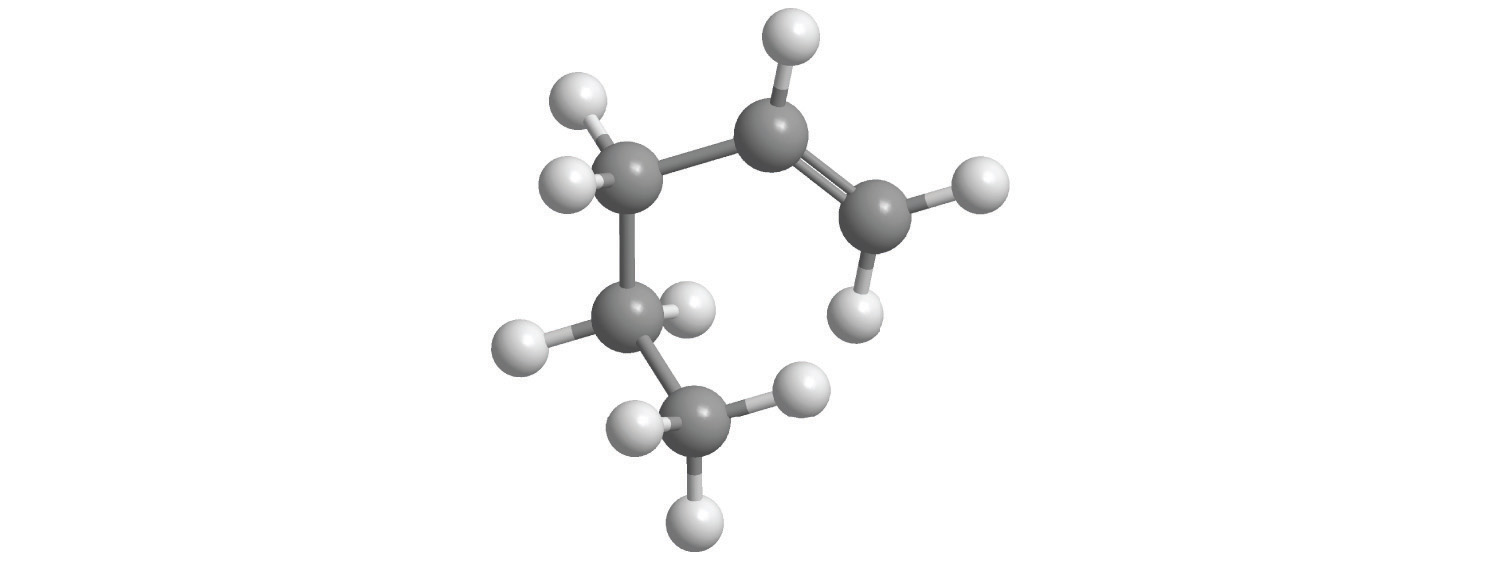5.5. Calculate the molar mass of each compound.

1.2.3.4.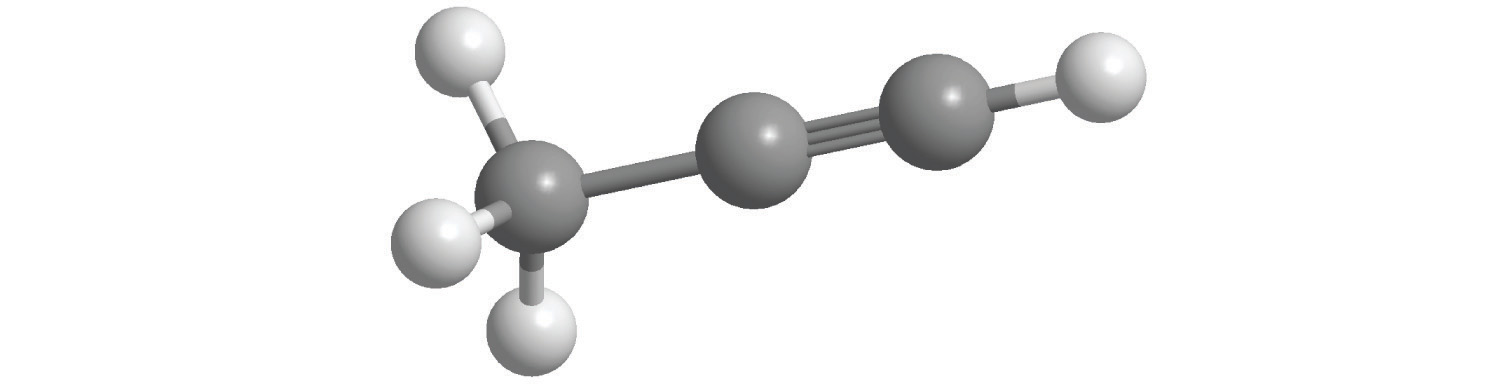6. For each compound, write the condensed formula, name the compound, and give its molar mass.

1.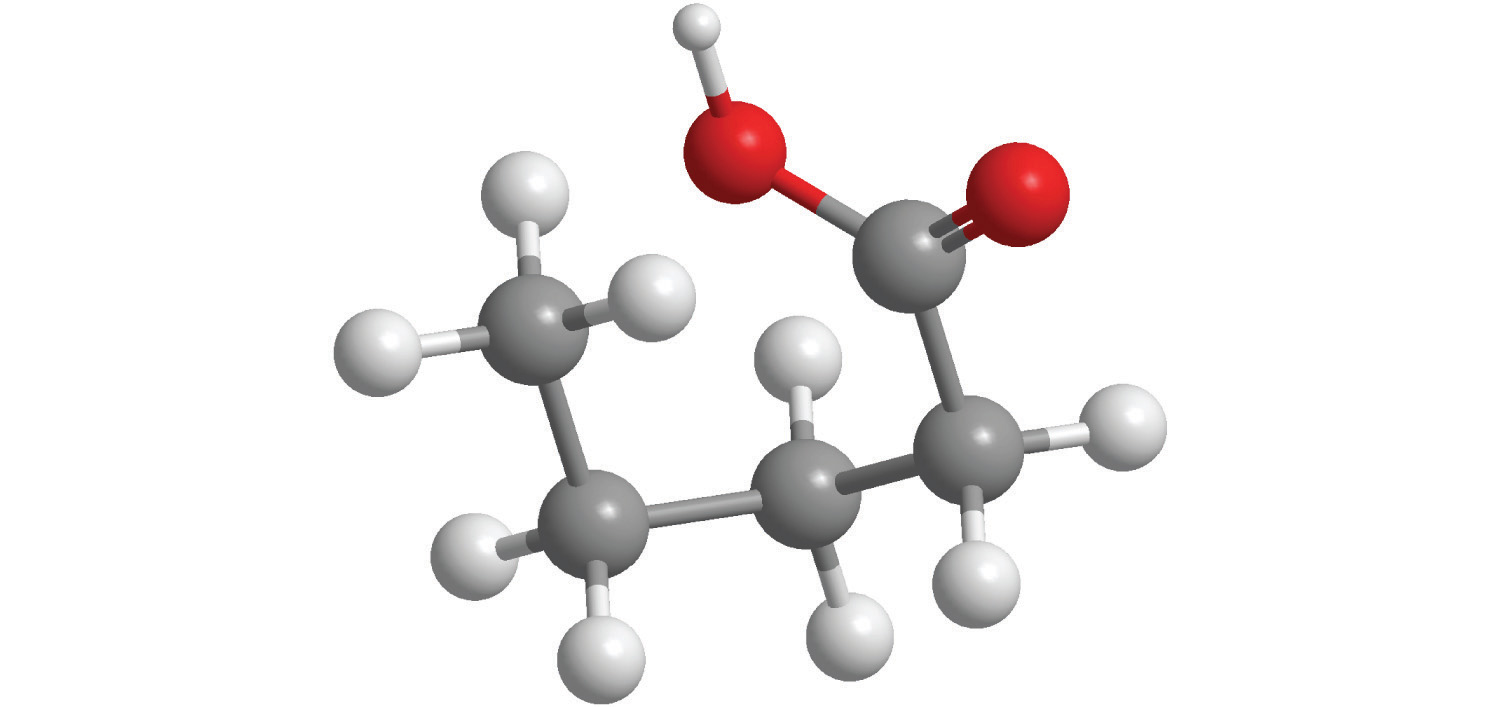2.7. For each compound, write the condensed formula, name the compound, and give its molar mass.

1.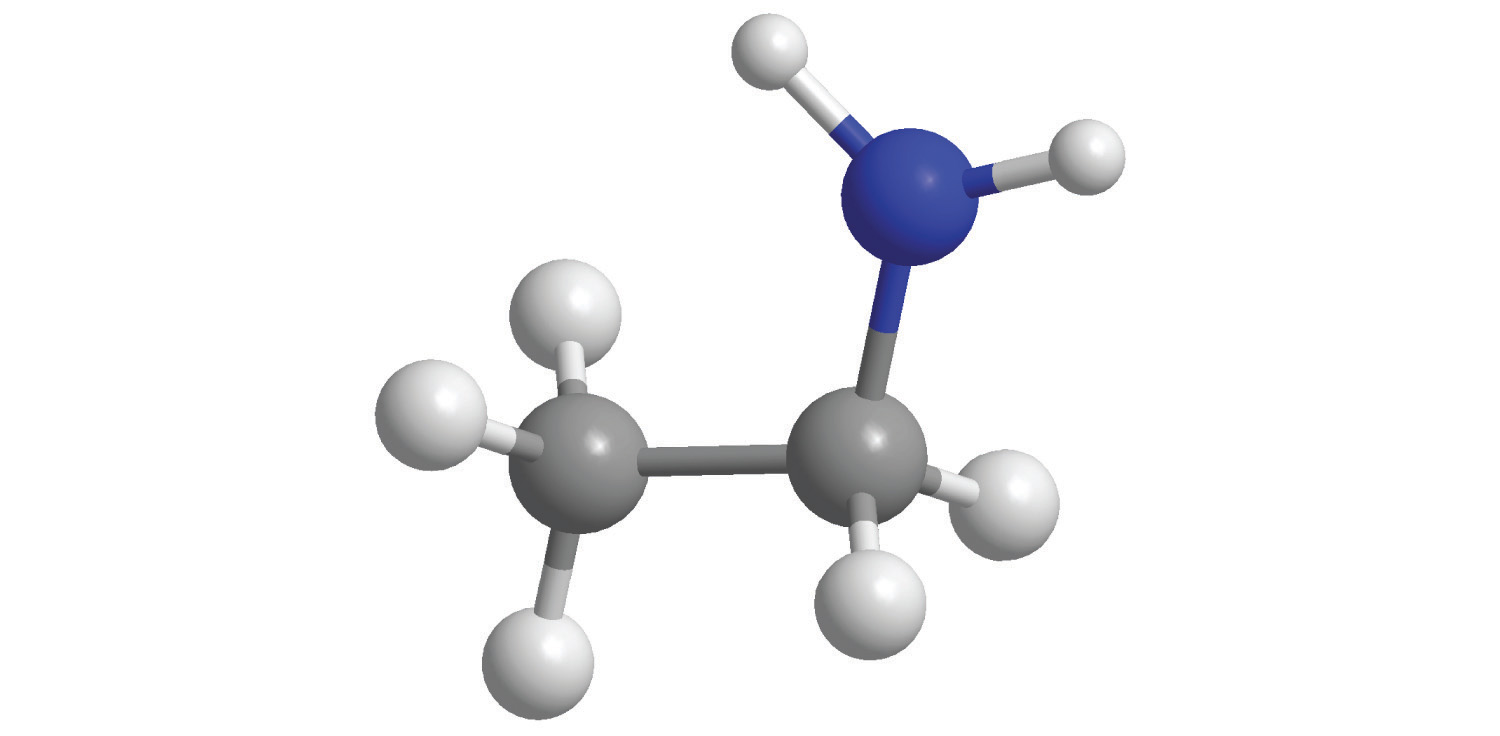2.8. Calculate the number of moles in 5.00 × 102 g of each substance. How many molecules or formula units are present in each sample?

1. CaO (lime)
2. CaCO3 (chalk)
3. C12H22O11 [sucrose (cane sugar)]
4. NaOCl (bleach)
5. CO2 (dry ice)
9. Calculate the mass in grams of each sample.

1. 0.520 mol of N2O4
2. 1.63 mol of C6H4Br2
3. 4.62 mol of (NH4)2SO3
10. Give the number of molecules or formula units in each sample.

1. 1.30 × 10−2 mol of SCl2
2. 1.03 mol of N2O5
3. 0.265 mol of Ag2Cr2O7
11. Give the number of moles in each sample.

1. 9.58 × 1026 molecules of Cl2
2. 3.62 × 1027 formula units of KCl
3. 6.94 × 1028 formula units of Fe(OH)2
12. Solutions of iodine are used as antiseptics and disinfectants. How many iodine atoms correspond to 11.0 g of molecular iodine (I2)?

13. What is the total number of atoms in each sample?

1. 0.431 mol of Li
2. 2.783 mol of methanol (CH3OH)
3. 0.0361 mol of CoCO3
4. 1.002 mol of SeBr2O
14. What is the total number of atoms in each sample?

1. 0.980 mol of Na
2. 2.35 mol of O2
3. 1.83 mol of Ag2S
4. 1.23 mol of propane (C3H8)
15. What is the total number of atoms in each sample?

1. 2.48 g of HBr
2. 4.77 g of CS2
3. 1.89 g of NaOH
4. 1.46 g of SrC2O4
16. Decide whether each statement is true or false and explain your reasoning.

1. There are more molecules in 0.5 mol of Cl2 than in 0.5 mol of H2.
2. One mole of H2 has 6.022 × 1023 hydrogen atoms.
3. The molecular mass of H2O is 18.0 amu.
4. The formula mass of benzene is 78 amu.
17. Complete the following table.

Substance Mass (g) Number of Moles Number of Molecules or Formula Units Number of Atoms or Ions
MgCl2 37.62
AgNO3 2.84
BH4Cl 8.93 × 1025
K2S 7.69 × 1026
H2SO4 1.29
C6H14 11.84
HClO3 2.45 × 1026
18. Give the formula mass or the molecular mass of each substance.

1. PbClF
2. Cu2P2O7
3. BiONO3
4. Tl2SeO4
19. Give the formula mass or the molecular mass of each substance.

1. MoCl5
2. B2O3
3. UO2CO3
4. NH4UO2AsO4